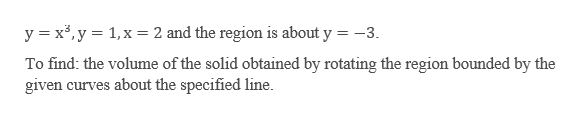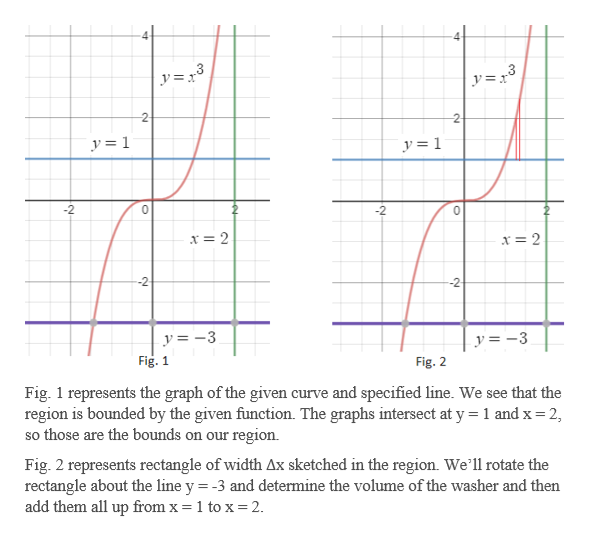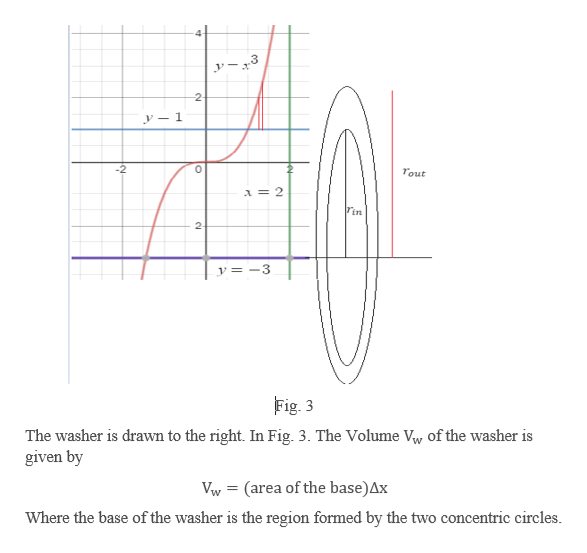# Find the volume of the solid obtained by rotating the region bounded by the given curves about the specified line. Sketch the region, the solid, and a typical disk or washer for y = x^3, y=1, x=2; about y=-3

Question

Find the volume of the solid obtained by rotating the region bounded by the given curves about the specified line. Sketch the region, the solid, and a typical disk or washer for y = x^3, y=1, x=2; about y=-3

check_circle

Step 1

Given a solid obtained by rotating the region bounded by the following curves:help_outlineImage Transcriptionclosey x3,y 1,x2 and the region is about y = -3 To find: the volume of the solid obtained by rotating the region bounded by the given curves about the specified line fullscreen
Step 2

We begin with sketching the graph of the given curves.help_outlineImage Transcriptionclosey= r° y=x° 2 2 y= 1 y= 1 -2 0 -2 0 x =2 x = 2 -2 y=3 y= -3 Fig. 1 Fig. 2 Fig. 1 represents the graph of the given curve and specified line. We see that the region is bounded by the given function. The graphs intersect at y 1 and x 2, so those are the bounds on our region Fig. 2 represents rectangle of width Ax sketched in the region. We'll rotate the rectangle about the line y = -3 and determine the volume of the washer and then add them all up from x = 1 to x=2 fullscreen
Step 3

To define washer in th...help_outlineImage Transcriptionclose2 y-1 -2 Tout A = 2 in 2 y= -3 Fig. 3 The washer is drawn to the right. In Fig. 3. The Volume Vw of the washer is given by Vw(area of the base)Ax Where the base of the washer is the region formed by the two concentric circles. fullscreen

### Want to see the full answer?

See Solution

#### Want to see this answer and more?

Solutions are written by subject experts who are available 24/7. Questions are typically answered within 1 hour.*

See Solution
*Response times may vary by subject and question.
Tagged in

### Integration